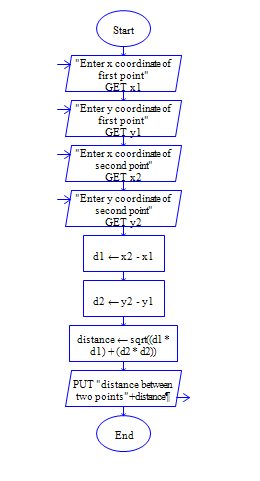# C Program to find the distance between two points in 2D space

Get x and y coordinates of two points(x1,y1,x2,y2) and find distance between two points

Sample Input 1:

3 4 4 5

Sample Output 1:

1.414

#### Flow Chart DesignStrongly recommended to Solve it on your own, Don't directly go to the solution given below.

#include<stdio.h> int main() { //write your code here }

#### Program or Solution

``` #include<stdio.h> #include<math.h> int main() { int x1,x2,y1,y2; int x,y; //temporary variables float distance; printf("Enter X and Y coordinates of first point"); scanf("%d %d",&x1,&y1); printf("Enter X and Y coordinates of Second point"); scanf("%d %d",&x2,&y2); x=x2-x1; y=y2-y1; distance=sqrt((x*x)+(y*y)); printf("Distance: %.2f",distance); return 0; } ```

#### Program Explanation

Get x1,y1,x2 and y2 coordinates of two points(using scanf statement)

Calculate distance by the eculdian distance formula.

Math.sqrt is function available in c library (in math.h header file)to find square root.

Print distance (using printf statement).